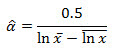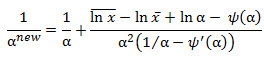## Fitting a gamma distribution in SQL Server

Apr 30

Written by: Charles Flock
4/30/2018 9:13 AMBased on the paper ‘Estimating a Gamma Distribution’ by Thomas P. Minka we look at a very quick technique in SQL Server to estimate the alpha and beta parameters of a gamma distribution based on sample data.

In applied statistics there are times when you want to take your sample data and estimate the parameters of the distribution that best describes it. Without going too deeply into the math we look at how to fit a gamma distribution to the data. Specifically, we are trying to find the alpha and beta parameters to pass to the GAMMADIST function to calculate the probability density function. Doing this on the database is interesting because 1) that’s where our data is and 2) it makes it easy to include the calculation in work flows where, for example, we might want to do predictive analytics. Plus, it’s interesting and pretty easy to do.

The focus of the fitting function is on the alpha (α) parameter as the beta (β) parameter can be calculated directly from α.

The initial estimate for α isThe update step iswhere ψ is the digamma function and ψ′ is the trigamma function.

Let’s look at our SQL Server implementation.

First, let’s declare some variables

DECLARE @tol as float = 0.00000001490116119384765625;
DECLARE @xbar as float;
DECLARE @logxbar as float;
DECLARE @meanlogx as float;
Use the XLeratorDB RANDGAMMA function to create some sample data and calculate x, ln x, and ln x.
SELECT
@xbar = AVG(x)
,@logxbar = LOG(AVG(x))
,@meanlogx = AVG(LOG(x))
FROM
(SELECT x as x FROM wct.RANDGAMMA(1000,5,3))n;

Use a recursive CTE to calculate the alpha parameter of the gamma distribution. In this CTE we want to keep track of the number of iterations, the value of alpha and an error term. The number of iterations starts at 0 and terminates at 100. The initial value of alpha is as described above. The error term is simply the absolute value of the change in alpha |α(n+1)n|. We will initialize the error term to 1 and then iterate until the error term is less than the tolerance (defined above) or we have reached the maximum number of iterations. The anchor part of our CTE looks like this:

SELECT
0 as iter
,0.5 / (@logxbar - @meanlogx) as alpha
,1e+00 as err

The recursive part of the CTE does three things. First, it increments the iter column by 1. Second, the alpha column incorporates the update equation described above using the XLeratorDB DIGAMMA and TRIGAMMA functions. Third, the err column is populated as the absolute value of the difference between the current alpha value and the alpha value from the previous iteration.

SELECT
n.iter + 1
,n.alpha1
,ABS(n.alpha1 - n.alpha0) as err
FROM (
SELECT iter, alpha, 1/(1/alpha + (@meanlogx - @logxbar + LOG(alpha) - wct.digamma(alpha))/(POWER(alpha,2)*(1/alpha-wct.trigamma(alpha))))
FROM x
WHERE err > @tol
Wrap this inside the CTE and select all the rows, and we get something that looks like this (your results will be different as the test data are randomly generated).
with x as (
SELECT
0 as iter
,0.5 / (@logxbar - @meanlogx) as alpha
,1e+00 as err
UNION ALL
SELECT
n.iter + 1
,n.alpha1
,ABS(n.alpha1 - n.alpha0) as err
FROM (
SELECT iter, alpha, 1/(1/alpha + (@meanlogx - @logxbar + LOG(alpha) - wct.digamma(alpha))/(POWER(alpha,2)*(1/alpha-wct.trigamma(alpha))))
FROM x
WHERE err > @tol
)n(iter, alpha0, alpha1)
)
SELECT
*
FROM
x
ORDER BY
iter ASC
OPTION
(MAXRECURSION 100);

This produces the following result.

You can see that the root-finding algorithm got to within tolerance very quickly. In the next SELECT statement, we generate a new sample of data and we return just the final estimates of alpha and beta and the number of iterations to arrive at a solution.

SELECT
@xbar = AVG(x)
,@logxbar = LOG(AVG(x))
,@meanlogx = AVG(LOG(x))
FROM
(SELECT x as x FROM wct.RANDGAMMA(1000,7,0.5))n;

with x as (
SELECT
0 as iter
,0.5 / (@logxbar - @meanlogx) as alpha
,1e+00 as err
UNION ALL
SELECT
n.iter + 1
,n.alpha1
,ABS(n.alpha1 - n.alpha0) as err
FROM (
SELECT iter, alpha, 1/(1/alpha + (@meanlogx - @logxbar + LOG(alpha) - wct.digamma(alpha))/(POWER(alpha,2)*(1/alpha-wct.trigamma(alpha))))
FROM x
WHERE err > @tol
)n(iter, alpha0, alpha1)
)
SELECT TOP 1
alpha
,@xbar / alpha as beta
,iter
FROM
x
ORDER BY
iter DESC
OPTION
(MAXRECURSION 100);

This produces the following result.

As you can see, the fitting algorithm came with an alpha parameter of 7.35 and a beta parameter 0.48 compared to values of 7.0 and 0.5 which were used to generate the sample data. Obviously, the larger the sample size, the better the fit will be.

In our testing the fit always happens in less than 5 iterations and in no measurable clock time. Your results will depend on your configuration, but this really isn’t a very expensive calculation at all.

If you are interested in doing other sophisticated calculations like this in SQL Server using simple SQL statements download the 15-day trial. If there are other calculations you would like to see or you have questions about XLeratorDB, please send an email to support@westclintech.com.

Tags:
Categories:
Location: BlogsThe WestClinTech Blog

## Search Blogs

 KeywordsPhrase

## Blog Archives

Archive
 < December 2023 >
SunMonTueWedThuFriSat
262728293012
3456789
10111213141516
17181920212223
24252627282930
31123456
Monthly
Go

### SupportCopyright 2008-2023 Westclintech LLC         Privacy Policy        Terms of Service